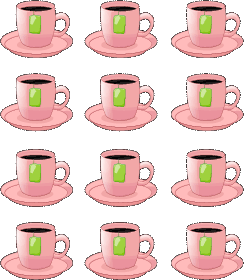# Identify Multiplication Expressions Using Arrays

## Arrays To Show Multiplication Concepts

Kids gain understanding and fluency when they understand multiplication in different ways. Apart from equal groups' method, we can also identify multiplication expressions using arrays. Unlike the case with equal groups where objects are arranged in groups of equal sizes, an array is arranged in a rectangular form of equal rows and equal columns.
This rectangular representation provide kids with a clear visual pattern of arrays to show multiplication concepts. Just like equal groups, with arrays, rows represent the number of groups, while columns represent the group size.

## Click the sections below to find more explanation on this topic

...

### Arrays - kids inspiration to quick identify multiplication expressions

In our test - identify multiplication expression using arrays, we have used very beautiful models to stimulate kids into a quick and concrete understanding of a multiplication expression.

When the structure of an array is given, we simply count the number of elements in the first column then we multiply by the number of elements on the first row.

### Example: use arrays to identify multiplication expression

Consider an array where each column represents a group of 4 cups and 3 cups in each row.From the given multiplication expressions, which multiplication expression describes this array?

• 4 x 6
• 4 x 12
• 4 x 3

'Bingo'! I got it. The correct answer is 4 x 3.

The first factor 4 in the multiplication expression represents 4 equal columns of groups of cups,
while the second factor 3 represents 3 equal rows of cups.